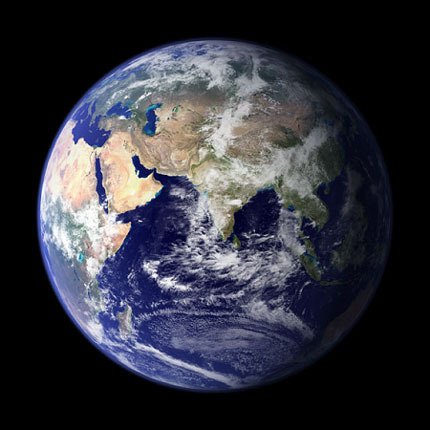•• # How Fast Does the Earth Spin?

One of the basic questions regarding the earth is, how fast does it spin? The earth is constantly spinning; it spins around its axis. An axis in this case is an imaginary line running through the center of the earth from the North Pole to the South Pole. To calculate how fast the earth spins, we need to know a few details.

To figure out the speed of the earth at the equator, we need to know the circumference of the earth at the equator. The circumference of the earth at the equator is 25, 040 miles (40,070 KM). The earth makes a full revolution once a day-about every 24 hours (an exact day is 23 hours 56 minutes 04. 09053 seconds). Once we know the circumference and time required for one revolution, we can simply divide 25040/24. The answer to how fast the earth spins is at the equator is 1,038 miles per hour (1674.66km/hr).

## The Earth's Speed Differs Depending on Location

It should be noted that the earth speed is not the same all over the globe; in fact the earth's speed can vary depending on the distance from the equator to the poles. For instance, the earth's speed is fastest at the equator- where the circumference has the most distance to travel around the axis. At the equator, the earth spins at 1,038 mph, as stated above, however if you are directly on the North or South Pole, the distance for the earth to revolve around the axis is practically zero meaning that the earth's speed is extremely slow. In fact, the earth's speed at the North or South Pole is about one centimeter per 24 hour period.If you live midway between the poles and the equator (e.g. New York or Europe), the earth still spins fast, but not as fast as it does at the equator. Speeds for these locations are approximately 700 to 900 mph (1125 to 1450 kph).

## Calculating the Speed of the Earth at Your Location

It is pretty easy to calculate the speed of the earth wherever you are located, all you need to know is the circumference of the earth at your location and divide it by 24 (the amount of hours in a day). To figure out the circumference of the earth at your location, you might want to have a calculator handy. You will need to multiply by the cosine of your latitude and then divide by 24.

The formula to figure out the circumference of the earth at a specific degree is as follows:

C = 2 pi r cos(x),

Pi = 3.14159

r = the earth's equatorial radius = 3963 miles (6378 km)

X = the angle of latitude

1. #### jordanlickscum

3 October, 2011 at 11:58 am

What’s the story of balamory?

2. #### glenn miller

25 May, 2011 at 5:58 am

This formula is wrong!
The true formula is;
diameter of earth in miles, multiply pi, divide this by 24 (hrs), multiply cosine of  degrees of latitude = mph